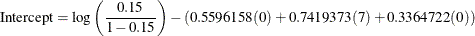### LOGISTIC Statement

LOGISTIC <options> ;

The LOGISTIC statement performs power and sample size analyses for the likelihood ratio chi-square test of a single predictor in binary logistic regression, possibly in the presence of one or more covariates that might be correlated with the tested predictor.

#### Summary of Options

Table 71.2 summarizes the options available in the LOGISTIC statement.

Table 71.2: LOGISTIC Statement Options

Option

Description

Define analysis

Specifies the statistical analysis

Specify analysis information

Specifies the significance level

Specifies the distributions of predictor variables

Specifies the distribution of the predictor variable being tested

Defines a distribution for a predictor variable

Specify effects

Specifies the multiple correlation between the predictor and the covariates

Specifies the odds ratios for the covariates

Specifies the regression coefficients for the covariates

Specifies the default change in the predictor variables

Specifies the intercept

Specifies the response probability

Specifies the odds ratio being tested

Specifies the regression coefficient for the predictor variable

Specifies the changes in the predictor variables

Specify sample size

Enables fractional input and output for sample sizes

Specifies the sample size

Specify power

Specifies the desired power of the test

Specify computational method

Specifies the default number of categories for each predictor variable

Specifies the number of categories for predictor variables

Control ordering in output

Controls the output order of parameters

Table 71.3 summarizes the valid result parameters in the LOGISTIC statement.

Table 71.3: Summary of Result Parameters in the LOGISTIC Statement

Analyses

Solve For

Syntax

TEST=LRCHI

Power

Sample size

#### Dictionary of Options

ALPHA=number-list

specifies the level of significance of the statistical test. The default is 0.05, corresponding to the usual 0.05100% = 5% level of significance. See the section Specifying Value Lists in Analysis Statements for information about specifying the number-list.

CORR=number-list

specifies the multiple correlation (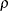) between the tested predictor and the covariates. If you also specify the COVARIATES= option, then the sample size is either multiplied (if you are computing power) or divided (if you are computing sample size) by a factor of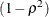. See the section Specifying Value Lists in Analysis Statements for information about specifying the number-list.

COVARIATES=grouped-name-list

specifies the distributions of any predictor variables in the model but not being tested, using labels specified with the VARDIST= option. The distributions are assumed to be independent of each other and of the tested predictor. If this option is omitted, then the tested predictor specified by the TESTEDPREDICTOR= option is assumed to be the only predictor in the model. See the section Specifying Value Lists in Analysis Statements for information about specifying the grouped-name-list.

COVODDSRATIOS=grouped-number-list

specifies the odds ratios for the covariates in the full model (including variables in the TESTPREDICTOR= and COVARIATES= options). The ordering of the values corresponds to the ordering in the COVARIATES= option. If the response variable is coded as Y = 1 for success and Y = 0 for failure, then the odds ratio for each covariate X is the odds of Y = 1 when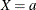divided by the odds of Y = 1 when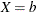, where a and b are determined from the DEFAULTUNIT= and UNITS= options. Values must be greater than zero. See the section Specifying Value Lists in Analysis Statements for information about specifying the grouped-number-list.

COVREGCOEFFS=grouped-number-list

specifies the regression coefficients for the covariates in the full model including the test predictor (as specified by the TESTPREDICTOR= option). The ordering of the values corresponds to the ordering in the COVARIATES= option. See the section Specifying Value Lists in Analysis Statements for information about specifying the grouped-number-list.

DEFAULTNBINS=number

specifies the default number of categories (or bins) into which the distribution for each predictor variable is divided in internal calculations. Higher values increase computational time and memory requirements but generally lead to more accurate results. Each test predictor or covariate that is absent from the NBINS= option derives its bin number from the DEFAULTNBINS= option. The default value is DEFAULTNBINS=10.

There are two variable distributions for which the number of bins can be overridden internally:

• For an ordinal distribution, the number of ordinal values is always used as the number of bins.

• For a binomial distribution, if the requested number of bins is larger than n + 1, where n is the sample size parameter of the binomial distribution, then exactly n + 1 bins are used.

DEFAULTUNIT=change-spec

specifies the default change in the predictor variables assumed for odds ratios specified with the COVODDSRATIOS= and TESTODDSRATIO= options. Each test predictor or covariate that is absent from the UNITS= option derives its change value from the DEFAULTUNIT= option. The value must be nonzero. The default value is DEFAULTUNIT=1. This option can be used only if at least one of the COVODDSRATIOS= and TESTODDSRATIO= options is used.

Valid specifications for change-spec are as follows:

number

defines the odds ratio as the ratio of the response variable odds whento the odds when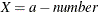for any constant a.

<+ | ->SD

defines the odds ratio as the ratio of the odds whento the odds when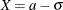(or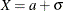, if SD is preceded by a minus sign (–)) for any constant a, where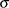is the standard deviation of X (as determined from the VARDIST= option).

multiple*SD

defines the odds ratio as the ratio of the odds whento the odds when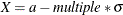for any constant a, whereis the standard deviation of X (as determined from the VARDIST= option).

PERCENTILES(p1, p2)

defines the odds ratio as the ratio of the odds when X is equal to its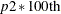percentile to the odds when X is equal to its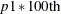percentile (where the percentiles are determined from the distribution specified in the VARDIST= option). Values for p1 and p2 must be strictly between 0 and 1.

INTERCEPT=number-list

specifies the intercept in the full model (including variables in the TESTPREDICTOR= and COVARIATES= options). See the section Specifying Value Lists in Analysis Statements for information about specifying the number-list.

NBINS=(name = number <…"name" = number>)

specifies the number of categories (or bins) into which the distribution for each predictor variable (identified by its name from the VARDIST= option) is divided in internal calculations. Higher values increase computational time and memory requirements but generally lead to more accurate results. Each predictor variable that is absent from the NBINS= option derives its bin number from the DEFAULTNBINS= option.

There are two variable distributions for which the NBINS= value can be overridden internally:

• For an ordinal distribution, the number of ordinal values is always used as the number of bins.

• For a binomial distribution, if the requested number of bins is larger than n + 1, where n is the sample size parameter of the binomial distribution, then exactly n + 1 bins are used.

NFRACTIONAL
NFRAC

enables fractional input and output for sample sizes. See the section Sample Size Adjustment Options for information about the ramifications of the presence (and absence) of the NFRACTIONAL option.

NTOTAL=number-list

specifies the sample size or requests a solution for the sample size with a missing value (NTOTAL=.). Values must be at least one. See the section Specifying Value Lists in Analysis Statements for information about specifying the number-list.

OUTPUTORDER=INTERNAL
OUTPUTORDER=REVERSE
OUTPUTORDER=SYNTAX

controls how the input and default analysis parameters are ordered in the output. OUTPUTORDER=INTERNAL (the default) arranges the parameters in the output according to the following order of their corresponding options:

The OUTPUTORDER=SYNTAX option arranges the parameters in the output in the same order in which their corresponding options are specified in the LOGISTIC statement. The OUTPUTORDER=REVERSE option arranges the parameters in the output in the reverse of the order in which their corresponding options are specified in the LOGISTIC statement.

POWER=number-list

specifies the desired power of the test or requests a solution for the power with a missing value (POWER=.). The power is expressed as a probability, a number between 0 and 1, rather than as a percentage. See the section Specifying Value Lists in Analysis Statements for information about specifying the number-list.

RESPONSEPROB=number-list

specifies the response probability in the full model when all predictor variables (including variables in the TESTPREDICTOR= and COVARIATES= options) are equal to their means. The log odds of this probability are equal to the intercept in the full model where all predictor are centered at their means. If the response variable is coded as Y = 1 for success and Y = 0 for failure, then this probability is equal to the mean of Y in the full model when all Xs are equal to their means. Values must be strictly between zero and one. See the section Specifying Value Lists in Analysis Statements for information about specifying the number-list.

TEST=LRCHI

specifies the likelihood ratio chi-square test of a single model parameter in binary logistic regression. This is the default test option.

TESTODDSRATIO=number-list

specifies the odds ratio for the predictor variable being tested in the full model (including variables in the TESTPREDICTOR= and COVARIATES= options). If the response variable is coded as Y = 1 for success and Y = 0 for failure, then the odds ratio for the X being tested is the odds of Y = 1 whendivided by the odds of Y = 1 when, where a and b are determined from the DEFAULTUNIT= and UNITS= options. Values must be greater than zero. See the section Specifying Value Lists in Analysis Statements for information about specifying the number-list.

TESTPREDICTOR=name-list

specifies the distribution of the predictor variable being tested, using labels specified with the VARDIST= option. This distribution is assumed to be independent of the distributions of the covariates as defined in the COVARIATES= option. See the section Specifying Value Lists in Analysis Statements for information about specifying the name-list.

TESTREGCOEFF=number-list

specifies the regression coefficient for the predictor variable being tested in the full model including the covariates specified by the COVARIATES= option. See the section Specifying Value Lists in Analysis Statements for information about specifying the number-list.

UNITS=(name = change-spec <…"name" = change-spec>)

specifies the changes in the predictor variables assumed for odds ratios specified with the COVODDSRATIOS= and TESTODDSRATIO= options. Each predictor variable whose name (from the VARDIST= option) is absent from the UNITS option derives its change value from the DEFAULTUNIT= option. This option can be used only if at least one of the COVODDSRATIOS= and TESTODDSRATIO= options is used.

Valid specifications for change-spec are as follows:

number

defines the odds ratio as the ratio of the response variable odds whento the odds whenfor any constant a.

<+ | ->SD

defines the odds ratio as the ratio of the odds whento the odds when(or, if SD is preceded by a minus sign (–)) for any constant a, whereis the standard deviation of X (as determined from the VARDIST= option).

multiple*SD

defines the odds ratio as the ratio of the odds whento the odds whenfor any constant a, whereis the standard deviation of X (as determined from the VARDIST= option).

PERCENTILES(p1, p2)

defines the odds ratio as the ratio of the odds when X is equal to itspercentile to the odds when X is equal to itspercentile (where the percentiles are determined from the distribution specified in the VARDIST= option). Values for p1 and p2 must be strictly between 0 and 1.

Each unit value must be nonzero.

VARDIST("label")=distribution (parameters)

defines a distribution for a predictor variable.

For the VARDIST= option,

label

identifies the variable distribution in the output and with the COVARIATES= and TESTPREDICTOR= options.

distribution

specifies the distributional form of the variable.

parameters

specifies one or more parameters associated with the distribution.

Choices for distributional forms and their parameters are as follows:

ORDINAL ((values) : (probabilities))

is an ordered categorical distribution. The values are any numbers separated by spaces. The probabilities are numbers between 0 and 1 (inclusive) separated by spaces. Their sum must be exactly 1. The number of probabilities must match the number of values.

BETA (a, b <, l, r >)

is a beta distribution with shape parameters a and b and optional location parameters l and r. The values of a and b must be greater than 0, and l must be less than r. The default values for l and r are 0 and 1, respectively.

BINOMIAL (p, n)

is a binomial distribution with probability of success p and number of independent Bernoulli trials n. The value of p must be greater than 0 and less than 1, and n must be an integer greater than 0. If n = 1, then the distribution is binary.

EXPONENTIAL ()

is an exponential distribution with scale, which must be greater than 0.

GAMMA (a,)

is a gamma distribution with shape a and scale. The values of a andmust be greater than 0.

LAPLACE (,)

is a Laplace distribution with locationand scale. The value ofmust be greater than 0.

LOGISTIC (,)

is a logistic distribution with locationand scale. The value ofmust be greater than 0.

LOGNORMAL (,)

is a lognormal distribution with locationand scale. The value ofmust be greater than 0.

NORMAL (,)

is a normal distribution with meanand standard deviation. The value ofmust be greater than 0.

POISSON (m)

is a Poisson distribution with mean m. The value of m must be greater than 0.

UNIFORM (l, r)

is a uniform distribution on the intervall, r, where l
r.

#### Restrictions on Option Combinations

To specify the intercept in the full model, choose one of the following two parameterizations:

• intercept (using the INTERCEPT= options)

• Prob(Y = 1) when all predictors are equal to their means (using the RESPONSEPROB= option)

To specify the effect associated with the predictor variable being tested, choose one of the following two parameterizations:

• odds ratio (using the TESTODDSRATIO= options)

• regression coefficient (using the TESTREGCOEFFS= option)

To describe the effects of the covariates in the full model, choose one of the following two parameterizations:

#### Option Groups for Common Analyses

This section summarizes the syntax for the common analyses supported in the LOGISTIC statement.

##### Likelihood Ratio Chi-Square Test for One Predictor

You can express effects in terms of response probability and odds ratios, as in the following statements:

proc power;
logistic
vardist("x1a") = normal(0, 2)
vardist("x1b") = normal(0, 3)
vardist("x2") = poisson(7)
vardist("x3a") = ordinal((-5 0 5) : (.3 .4 .3))
vardist("x3b") = ordinal((-5 0 5) : (.4 .3 .3))
testpredictor = "x1a" "x1b"
covariates = "x2" | "x3a" "x3b"
responseprob = 0.15
testoddsratio = 1.75
covoddsratios = (2.1 1.4)
ntotal = 100
power = .;
run;


The VARDIST= options define the distributions of the predictor variables. The TESTPREDICTOR= option specifies two scenarios for the test predictor distribution, Normal(10,2) and Normal(10,3). The COVARIATES= option specifies two covariates, the first with a Poisson(7) distribution. The second covariate has an ordinal distribution on the values –5, 0, and 5 with two scenarios for the associated probabilities: (.3, .4, .3) and (.4, .3, .3). The response probability in the full model with all variables equal to zero is specified by the RESPONSEPROB= option as 0.15. The odds ratio for a unit decrease in the tested predictor is specified by the TESTODDSRATIO= option to be 1.75. Corresponding odds ratios for the two covariates in the full model are specified by the COVODDSRATIOS= option to be 2.1 and 1.4. The POWER=. option requests a solution for the power at a sample size of 100 as specified by the NTOTAL= option.

Default values of the TEST= and ALPHA= options specify a likelihood ratio test of the first predictor with a significance level of 0.05. The default of DEFAULTUNIT=1 specifies that all odds ratios are defined in terms of unit changes in predictors. The default of DEFAULTNBINS=10 specifies that each of the three predictor variables is discretized into a distribution with 10 categories in internal calculations.

You can also express effects in terms of regression coefficients, as in the following statements:

proc power;
logistic
vardist("x1a") = normal(0, 2)
vardist("x1b") = normal(0, 3)
vardist("x2") = poisson(7)
vardist("x3a") = ordinal((-5 0 5) : (.3 .4 .3))
vardist("x3b") = ordinal((-5 0 5) : (.4 .3 .3))
testpredictor = "x1a" "x1b"
covariates = "x2" | "x3a" "x3b"
intercept = -6.928162
testregcoeff = 0.5596158
covregcoeffs = (0.7419373 0.3364722)
ntotal = 100
power = .;
run;


The regression coefficients for the tested predictor (TESTREGCOEFF=0.5596158) and covariates (COVREGCOEFFS=(0.7419373 0.3364722)) are determined by taking the logarithm of the corresponding odds ratios. The intercept in the full model is specified as –6.928162 by the INTERCEPT= option. This number is calculated according to the formula at the end of Analyses in the LOGISTIC Statement, which expresses the intercept in terms of the response probability, regression coefficients, and predictor means: